# The dam

The water reservoir is filled with first tributary for 25 hours second tributary for 35 hours. Some time were both inlets open, then a second tributary closed and the tank was filled in four hours. What time water flowed from both tributaries? (Expressed in hours and minutes)

Result

t = 12:55 hh:mm### Step-by-step explanation:

1/25 t + 1/35 (t+4) = 1

1/25•t + 1/35•(t+4) = 1

12t = 155

t = 155/12 ≈ 12.916667

Our simple equation calculator calculates it.Did you find an error or inaccuracy? Feel free to write us. Thank you!Tips to related online calculators
Looking for calculator of harmonic mean?
Looking for a statistical calculator?
Do you have a linear equation or system of equations and looking for its solution? Or do you have a quadratic equation?
Tip: Our volume units converter will help you with the conversion of volume units.
Do you want to convert time units like minutes to seconds?

## Related math problems and questions:

• Tributaries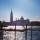The first tributary fill pool with water in 15 hours. The second tributary fill pool in 10 hours. For how many hours the pool is filled with both tributaries?
• Water reservoir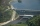One drain hole emptied the water from the tank for 20 hours, with the second drain for 30 hours. How long will the water drain from this tank if we open two drains simultaneously?
• Water inlets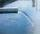Inlet valve with a flow rate of 12 liters per second is filled tank for 72 minutes. How long take to fill full tank if we open one more such valve half an hour after?
• Five inlets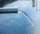The tank can be filled with five equally powerful inlets. If the tank is filled by four of these inlets, it takes a total of 30 minutes to fill one-third of the tank. How many minutes does it take to fill an empty tank if it is filled with all five inlets
• Two tributaries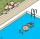Two tributaries of the pool fill it in 10 hours. One of the tributaries would fill 15 hours. How long would fill the first tributary?
• Water reservoirThe water reservoir is filled with one pump for 4 days by the second one for 9 days. Outlet can drain tank in 12 days. How long take fill the reservoir if both pumps are running and not closed outlet channel.
• Tank No 8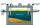Tank is filled by one inlet valve with a flow rate of 12 liters per second in 72 minutes. How long take the tank to fill, if we open half an hour after one more inlet?
• In and out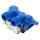The empty tank is filled in 12 minutes and empty in 16 minutes. How long does it take to fill if we forgot to close the drain? If the tank has 1000 l.
• Two pumps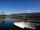The first pump fills the tank in 24 hours second pump in another 40 hours. For how long will it take to fill the tank if it operates both pumps at the same time?
• Three tributaries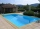It is possible to fill the pool with three tributaries. The first would take 12 hours, the second 15 hours, and the third 20 hours. The day before the summer season began, the manager opened all three tributaries simultaneously. How long did it take to fi
• Tank 9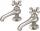The tank with volume V is filled with one pump for three hours and by the second pump for 5 hours. When both pumps run simultaneously, calculate: a) how much of the total volume of the tank is filled in one hour b) for how long is the tank full
• Oil tank and pipes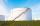The underground oil tank can be filled by two oil pipelines. The first is filled in 72 hours and the second in 48 hours. How many hours from the moment when first pipeline began to fill the oil is it necessary to start filling it with the second to fill i
• The tank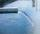The tank had 9 inflows to be filled in 21 days. After 9 days, 3 trips out. How many days did the remaining 6 tributaries fill the tank?
• Water reservoir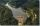The water reservoir is filled through one inlet 4 hours later than both together, then another inlet 9 hours later. For how long is filled by each separately?
• PumpingThe tank is filled with a larger pump in 12 hours and with scond pump in 15 hours. For how long does the tank fill when both pumps are switched on at the same time?
• Three pumps together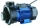One pump fills the tank in 1.5 hours, the second in 2 hours, and the third in 3 hours 20 minutes. How many minutes will the tank fill with three pumps if they work simultaneously?
• Pool 2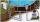The first supply by the pool fill for five hours and the second fill for six hours, drain should be drained for 15 hours. For how many hours the pool is full, when we open both inlet now and outlet open two hours later?